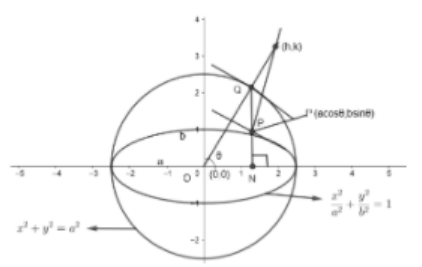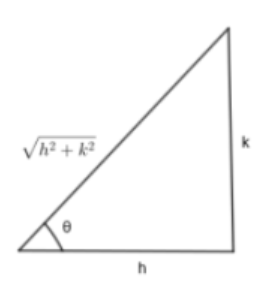QuestionAnswers

# Any ordinate $NP$ of an ellipse meets the auxiliary circle in $Q$; prove that the locus of the intersection of the normals at $P$ and $Q$ is the circle ${{x}^{2}}+{{y}^{2}}={{\left( a+b \right)}^{2}}$.Verified
149.1k+ views
Hint: Write the equation of the normals to ellipse and circle and satisfy $\left( h,k \right)$ in them.

We are given an ellipse with ordinate $NP$ which meets the auxiliary circle at $Q$.
We have to prove the locus of intersection of normals at $P$ and $Q$ is the circle ${{x}^{2}}+{{y}^{2}}={{\left( a+b \right)}^{2}}$Let $\left( h,k \right)$ be the point at which normals at $P$ and $Q$ meet. Here, $NP$ is ordinary which intersects the auxiliary circle at $Q$.
Let the ellipse be $\dfrac{{{x}^{2}}}{{{a}^{2}}}+\dfrac{{{y}^{2}}}{{{b}^{2}}}=1$
Since, $'a'$ is the radius of the auxiliary circle with a center $\left( 0,0 \right)$.
Therefore, we get the equation of the auxiliary circle as –
${{x}^{2}}+{{y}^{2}}={{a}^{2}}$
We know that any general point $P$ on the ellipse is given by $\left( x,y \right)=\left( a\cos \theta ,b\sin \theta \right)$
Also, we know that normal of the ellipse at a point $P\left( a\cos \theta ,b\sin \theta \right)$ is given by
$\dfrac{ax}{\cos \theta }-\dfrac{by}{\sin \theta }={{a}^{2}}-{{b}^{2}}$
Since, this normal passes through $\left( h,k \right)$, then
We get, $\dfrac{ah}{\cos \theta }-\dfrac{bk}{\sin \theta }={{a}^{2}}-{{b}^{2}}.....\left( i \right)$
We know the normal to the circle always passes through its center.
Therefore, normal at a point $Q$ will pass through the center of the auxiliary circle $\left( 0,0 \right)$. So the normal at $Q$ is $OQ$ which makes an angle $\theta$ with the $x$ axis.
We know that equation of any line passing through $\left( 0,0 \right)$ and the slope $'m'$ is given by
$\Rightarrow y=mx$
Therefore, the equation of $OQ$ is also
$\Rightarrow y=mx$
Since $NP$ is ordinate which meets the auxiliary circle at $Q$. Therefore, $OQ$ will make an angle $\theta$ with the $x$ axis.
So, $m=\tan \theta$
Hence, we get the equation of $OQ$ as
$\Rightarrow y=\left( \tan \theta \right)x$
$OQ$ also passes through $P\left( h,k \right)$, then
$\Rightarrow k=\left( \tan \theta \right)h$
Or, $\tan \theta =\dfrac{k}{h}$Therefore, we get $\sin \theta =\dfrac{k}{\sqrt{{{h}^{2}}+{{k}^{2}}}}$ and $\cos \theta =\dfrac{h}{\sqrt{{{h}^{2}}+{{k}^{2}}}}$
Putting the values of $\sin \theta$ and $\cos \theta$ in equation $\left( i \right)$, we get
$\dfrac{ah\left( \sqrt{{{h}^{2}}+{{k}^{2}}} \right)}{h}-\dfrac{bk\left( \sqrt{{{h}^{2}}+{{k}^{2}}} \right)}{k}={{a}^{2}}-{{b}^{2}}$
$\Rightarrow a\left( \sqrt{{{h}^{2}}+{{k}^{2}}} \right)-b\left( \sqrt{{{h}^{2}}+{{k}^{2}}} \right)={{a}^{2}}-{{b}^{2}}$
By taking $\sqrt{{{h}^{2}}+{{k}^{2}}}$ common, we get
$\Rightarrow \left( \sqrt{{{h}^{2}}+{{k}^{2}}} \right)\left( a-b \right)={{a}^{2}}-{{b}^{2}}$
$\Rightarrow \left( \sqrt{{{h}^{2}}+{{k}^{2}}} \right)=\dfrac{\left( {{a}^{2}}-{{b}^{2}} \right)}{\left( a-b \right)}$
Since we know that ${{x}^{2}}-{{y}^{2}}=\left( x-y \right)\left( x+y \right)$
We get, $\Rightarrow \left( \sqrt{{{h}^{2}}+{{k}^{2}}} \right)=\dfrac{\left( a-b \right)\left( a+b \right)}{\left( a-b \right)}$
We get, $\sqrt{{{h}^{2}}+{{k}^{2}}}=\left( a+b \right)$
By squaring both sides, we get
$\Rightarrow {{h}^{2}}+{{k}^{2}}={{\left( a+b \right)}^{2}}$
To get the locus, replace $h$ and $k$ by $x$ and $y$ respectively.
$\Rightarrow {{x}^{2}}+{{y}^{2}}={{\left( a+b \right)}^{2}}$
Hence Proved

Note: In questions involving ellipse, always take the general points in terms of $\theta$ to easily solve the questions. Note the normal to circle always passes through its center.